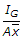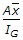# Mechanical Engineering - Hydraulics and Fluid Mechanics

### Exercise :: Hydraulics and Fluid Mechanics - Section 6

36.

The mercury does not wet the glass. This is due to the property of the liquid known as

 A. cohesion B. adhesion C. viscosity D. surface tension

Answer: Option D

Explanation:

No answer description available for this question. Let us discuss.

37.

The coefficient of discharge in case of internal mouthpiece is __________ that of external mouthpiece.

 A. less than B. more than

Answer: Option A

Explanation:

No answer description available for this question. Let us discuss.

38.

The centre of pressure for a vertically immersed surface lies at a distance equal to __________ the centre of gravity.

 A.below B.above C.below D.above

Answer: Option A

Explanation:

No answer description available for this question. Let us discuss.

39.

According to Manning's formula, the discharge through an open channel is (where M = Manning's constant)

 A. A x M x m1/2 x i2/3 B. A x M x m2/3 x i1/2 C. A1/2 x M2/3 x m x i D. A2/3 x M1/3 x m x i

Answer: Option B

Explanation:

No answer description available for this question. Let us discuss.

40.

When the venturimeter is inclined, then for a given flow it will show __________ reading.

 A. same B. more C. less

Answer: Option A

Explanation:

No answer description available for this question. Let us discuss.

#### Current Affairs 2021

Interview Questions and Answers